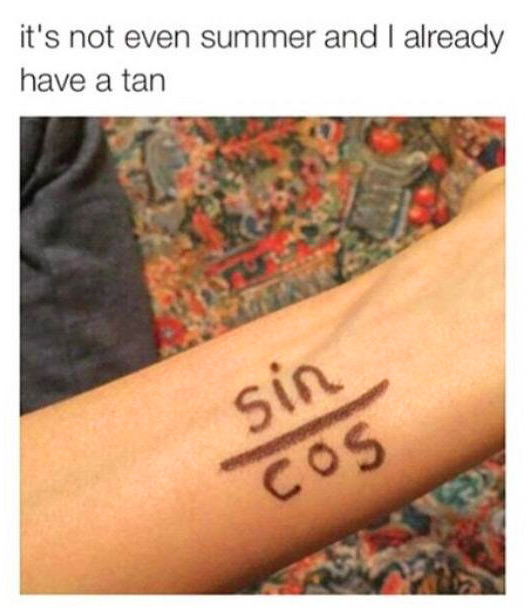Most who have taken high school math know that the three main functions in trigonometry are Sine, Cosine and Tangent. In a right triangle ABC, whose right angle is A, the tangent ratio of the angle B is the opposite leg over the adjacent leg. Therefore, in a right angle triangle, the tangent ratio of an angle, except the right angle, is: tan = sin / cos. Now this person decided to turn this equation into a pun. Click here for a few bonus nerdy jokes.

### 12. Bye-ology### 11. Be Rational### 10. Mole### 9. My Toe Sis### 8. Velociraptor### 7. So Oxygen### 6. You Are Acute### 5. I’m Traveling Light### 4. Word### 3. Water### 2. Foiled### 1. Ate Too Much Pi